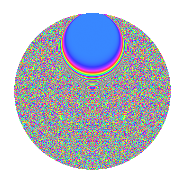# Properties

 Label 1089.2.bcLevel 1089 Weight 2 Character orbit bc Rep. character $$\chi_{1089}(4,\cdot)$$ Character field $$\Q(\zeta_{165})$$ Dimension 10400 Sturm bound 264

# Related objects

## Defining parameters

 Level: $$N$$ = $$1089 = 3^{2} \cdot 11^{2}$$ Weight: $$k$$ = $$2$$ Character orbit: $$[\chi]$$ = 1089.bc (of order $$165$$ and degree $$80$$) Character conductor: $$\operatorname{cond}(\chi)$$ = $$1089$$ Character field: $$\Q(\zeta_{165})$$ Sturm bound: $$264$$

## Dimensions

The following table gives the dimensions of various subspaces of $$M_{2}(1089, [\chi])$$.

Total New Old
Modular forms 10720 10720 0
Cusp forms 10400 10400 0
Eisenstein series 320 320 0

## Trace form

 $$10400q - 39q^{2} - 58q^{3} - 169q^{4} - 41q^{5} - 103q^{6} - 41q^{7} - 168q^{8} - 42q^{9} + O(q^{10})$$ $$10400q - 39q^{2} - 58q^{3} - 169q^{4} - 41q^{5} - 103q^{6} - 41q^{7} - 168q^{8} - 42q^{9} - 136q^{10} - 43q^{11} - 41q^{12} - 41q^{13} - 33q^{14} - 137q^{15} - 169q^{16} - 168q^{17} - 86q^{18} - 182q^{19} - 19q^{20} - 105q^{21} - 39q^{22} - 42q^{23} - 107q^{24} - 163q^{25} - 148q^{26} - 85q^{27} - 140q^{28} - 12q^{29} - 34q^{30} - 41q^{31} - 92q^{32} - 149q^{33} - 46q^{34} - 206q^{35} - 143q^{36} - 164q^{37} + 37q^{38} - 86q^{39} - 20q^{40} - 57q^{41} - 180q^{42} - 42q^{43} - 166q^{44} - 115q^{45} - 176q^{46} - 29q^{47} - 61q^{48} - 159q^{49} - 20q^{50} - 25q^{51} - 29q^{52} - 316q^{53} + 110q^{54} - 162q^{55} - 66q^{56} - 81q^{57} - 51q^{58} - 30q^{59} - 72q^{60} - 41q^{61} - 366q^{62} - 213q^{63} + 72q^{64} - 96q^{65} - 237q^{66} - 42q^{67} - 12q^{68} - 160q^{69} + 8q^{70} - 180q^{71} + 47q^{72} - 128q^{73} - 125q^{74} - 144q^{75} + 45q^{76} + 125q^{77} - 6q^{78} - 41q^{79} + 424q^{80} - 102q^{81} - 174q^{82} - 34q^{83} - 25q^{84} + 3q^{85} + 20q^{86} - q^{87} - 238q^{89} + 308q^{90} - 304q^{91} + 61q^{92} - 99q^{93} + 2q^{94} + 125q^{95} - 24q^{96} - 14q^{97} - 96q^{98} - 2q^{99} + O(q^{100})$$

## Decomposition of $$S_{2}^{\mathrm{new}}(1089, [\chi])$$ into newform subspaces

The newforms in this space have not yet been added to the LMFDB.

## Hecke Characteristic Polynomials

There are no characteristic polynomials of Hecke operators in the database